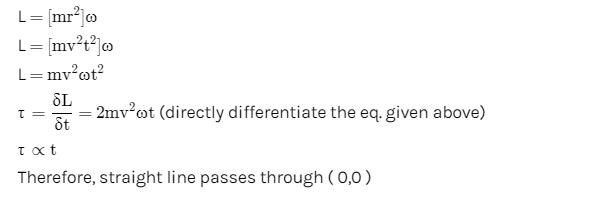Courses

# Previous Year Questions: Rotational Motion

## 22 Questions MCQ Test Physics For JEE | Previous Year Questions: Rotational Motion

Description
This mock test of Previous Year Questions: Rotational Motion for JEE helps you for every JEE entrance exam. This contains 22 Multiple Choice Questions for JEE Previous Year Questions: Rotational Motion (mcq) to study with solutions a complete question bank. The solved questions answers in this Previous Year Questions: Rotational Motion quiz give you a good mix of easy questions and tough questions. JEE students definitely take this Previous Year Questions: Rotational Motion exercise for a better result in the exam. You can find other Previous Year Questions: Rotational Motion extra questions, long questions & short questions for JEE on EduRev as well by searching above.
QUESTION: 1

### Three particles A, B and C, each of mass m, are connected to each other by three massless rigid rods to form a rigid, equilateral triangular body of side l. This body is placed on a horizontal frictionless table (x-y plane) and is hinged to it at the point A so that it can move without friction about the vertical axis through A (see figure). The body is set into rotational motion on the table about A with a constant angular velocity w.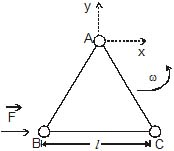[JEE'(Scr) 2002] (a) Find the magnitude of the horizontal force exerted by the hinge on the body (b) At time T, when the side BC is parallel to the x-axis, a force F is applied on B along BC (as shown). Obtain the x-component and the y-component of the force exterted by the hinge on the body, immediately after time T.

Solution:

ATQ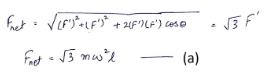Now,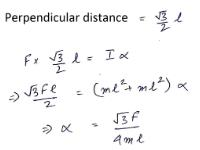So,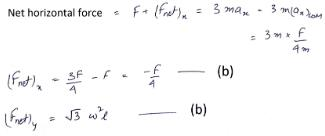Hence the answers should be changed to the ones in the solution marked as (a) and (b)

QUESTION: 2

### A particle is moving in a horizontal uniform circular motion. The angular momentum of the particle is conserved about the point :   [JEE'(Scr) 2003]

Solution:

The net force acting on a particle undergoing uniform circular motion is centripetal force which always passes through the centre of the circle. The torque due to this force about the centre is zero, therefore,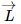is conserved about O.

QUESTION: 3

### Two particles each of mass M are connected by a massless rod of length l. The rod is lying on the smooth surface. If one of the particle is given an impulse MV as shown in the figure then angular velocity of the rod would be [JEE'(Scr) 2003]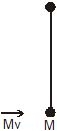Solution: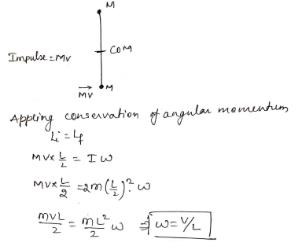QUESTION: 4

A disc is rolling (without slipping) on a horizontal surface. C is its center and Q and P are two points equidistant from C. Let Vp, VQ and VC be the magnitude of velocities of points P, Q and C respectively, then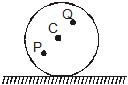[JEE'(Scr) 2004]

Solution:
QUESTION: 5

A child is standing with folded hands at the centre of a platform rotating about its central axis. The kinetic energy of the system is K. The child now stretches his arms so that the moment of inertia of the system doubles. The kinetic energy of the system now is

[JEE'(Scr) 2004]

Solution:
QUESTION: 6

A block of mass m is held fixed against a wall by a applying a hor izontal force F. Which of the following option is incorrect :

[JEE'(Scr) 2005]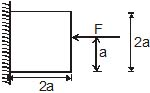Solution:

A. It is correct.
B. As F passes through centre torque will be 0.
C. Since the frictional force produces a torque, so, to balance it out normal force will also produce a torque.
D. It is also correct.

QUESTION: 7

A disc has mass 9M. A hole of radius R/3 is cut from it as shown in the figure. The moment of inertia of remaining part about an axis passing through the centre `O' of the disc and perpendicular to the plane of the disc is :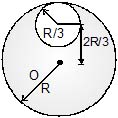[JEE'(Scr) 2005]

Solution:

The moment of inertia of complete disc about a perpendicular axis passing through center O is,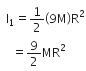The mass of cut out disc if radius R/3 is,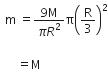Now, using the theorem of parallel axis, the moment of inertia of cut out disc about the perpendicular axis passing through center O is,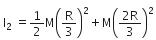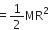The moment of inertia of residue disc is,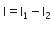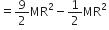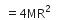QUESTION: 8

A particle moves in circular path with decreasing speed. Which of the following is correct

Solution:

Only the direction of L is constant because L = m v x r

QUESTION: 9

A solid sphere of mass M, radius R and having moment of inertia about an axis passing through the centre of mass as I, is recast into a disc of thickness t, whose moment of inertia about an axis passing through its edge and perpendicular to its plane remains I. Then, radius of the disc will be

[JEE' 2006]

Solution:
*Multiple options can be correct
QUESTION: 10

A solid cylinder of mass m and radius r is rolling on a rough inclined plane of inclination q. The coefficient of friction between the cylinder and incline is m. Then

[JEE' 2006]

Solution:

Fnet = mgsinθ – f
And Torque = F x R
So, if θ is decreased then torque is reduced and resultantly angular acceleration is reduced. Clearly, friction is opposing the translation and supports rotation.

*Multiple options can be correct
QUESTION: 11

A ball moves over a fixed track as shown in the figure. From A to B the ball rolls without slipping. Surface BC is frictionless. KA, KB and KC are kinetic energies of the ball at A, B and C, respe0ctively. Then

[JEE' 2006]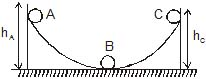Solution: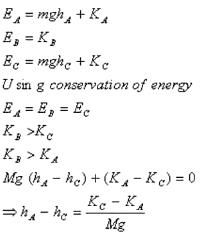QUESTION: 12

There is a rectangular plate of mass M kg of dimensions (a × b). The plate is held in horizontal position by striking n small balls each of mass m per unit area per unit time. These are striking in the shaded half region of the plate. The balls are colliding elastically with velocity v. What is v ?

[JEE' 2006]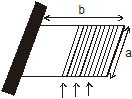It is given n = 100, M = 3 kg, m = 0.01 kg; b = 2 m, a = 1m; g = 10 m/s2.

Solution: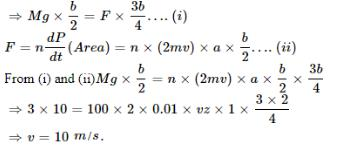QUESTION: 13

Two discs A and B are mounted coaxially on a vertical axle. The discs have moments of inertia I and 2I respectively about the common axis. Disc A is imparted an initial angular velocity 2w using the entire potential energy of a spring compressed by a distance x1. Disc B is imparted an angular velocity w by a spring having the same spring constant and compressed by a distance x2. Both the discs rotate in the clockwise direction.

The ratio x1/x2 is

[JEE' 2007]

Solution:
QUESTION: 14

Two discs A and B are mounted coaxially on a vertical axle. The discs have moments of inertia I and 2I respectively about the common axis. Disc A is imparted an initial angular velocity 2w using the entire potential energy of a spring compressed by a distance x1. Disc B is imparted an angular velocity w by a spring having the same spring constant and compressed by a distance x2. Both the discs rotate in the clockwise direction.

When disc B is brought in contact with disc A, they acquire a common angular velocity in time t. The average frictional torque on one disc by the other during this period is

[JEE' 2007]

Solution:
QUESTION: 15

Two discs A and B are mounted coaxially on a vertical axle. The discs have moments of inertia I and 2I respectively about the common axis. Disc A is imparted an initial angular velocity 2w using the entire potential energy of a spring compressed by a distance x1. Disc B is imparted an angular velocity w by a spring having the same spring constant and compressed by a distance x2. Both the discs rotate in the clockwise direction.

The loss of kinetic energy during the above process is

[JEE' 2007]

Solution:
QUESTION: 16

A small object of uniform density rolls up a curved surface with an initial velocity v. It reaches up to a maximum height of 3v2 / (4g) with respect to the initial position. The object is

[JEE' 2007]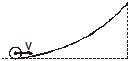Solution:
QUESTION: 17

STATEMENT-1 If there is no external torque on a body about its center of mass, then the velocity of the center of mass remains constant

because

STATEMENT-2 The linear momentum of an isolated system remains constant.

[JEE 2007]

Solution:

The true statements are

1. If there is no external torque on a body about its center of mass, then the angular velocity of the center of mass remains constant
2. The linear momentum of an isolated system remains constant.

QUESTION: 18

STATEMENT-1

Two cylinders, one hollow (metal) and the other solid (wood) with the same mass and identical dimensions are simultaneously allowed to roll without slipping down an inclined plane from the same height. The hollow cylinder will reach the bottom of the inclined plane first.

STATEMENT-2

By the principle of conservation of energy, the total kinetic energies of both the cylinders are identical when they reach the bottom of the incline.

[JEE-2008]

Solution:
QUESTION: 19

If the resultant of all the external forces acting on a system of particles is zero, then from an inertial frame, one can surely say that

[JEE 2009]

Solution:
*Multiple options can be correct
QUESTION: 20

A sphere is rolling without slipping on a fixed horizontal plane surface. In the figure is the point of contact, is the centre of the sphere and is its topmost point Then,

[JEE 2009]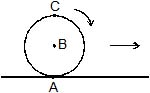Solution:
QUESTION: 21

A boy is pushing a ring of mass 2 kg and radius 0.5 m with a stick as shown in the figure. The stick applies a force of 2 N on the ring and rolls it without slipping with an acceleration of 0.3 m/s2. The coefficient of friction between the ground and ring is large enough that rolling always occurs and the coefficient of friction between the stick and the ring is (P/10). The value of P is?

[JEE 2011]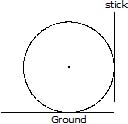Solution:

Let the friction between ground and sphere be fg
Let the friction between stick and sphere be fs​
Let the N be the normal reaction between sphere and stick
By 2nd law of motion
N−fg​= ma and N= 2Newton,
fg​= 1.4Newton
Torque about center is written by τ= Iα and τ= F x r
Hence (fg​−fs) r=Iα
Also, a= αr and I=mr2
On solving fs​=0.8=μN
μ= 0.4= 4/10 therefore P= 4.

QUESTION: 22

A thin uniform rod, pivoted at O is rotating in the horizontal plane with constant angular speed w, as shown in the figure. At time t = 0, small insect starts from O and moves with constant speed n with respect to the rod towards the other end. it reaches the end of the rod at t = T and stops. The angular speed of the system remains w throughout. The magnitude of the torque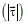on the system about O, as a function of time is best represented by which plot?

[JEE 2012]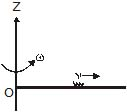Solution: